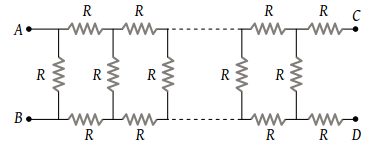NEET Physics Current Electricity Questions Solved

In the figure, the value of resistors to be connected between C and D so that the resistance of the entire circuit between A and B does not change with the number of elementary sets used is(1) R

(2) $R\left(\sqrt{3}-1\right)$

(3) 3 R

(4) $R\left(\sqrt{3}+1\right)$

Explanation is a part of a Paid Course. To view Explanation Please buy the course.

Difficulty Level: